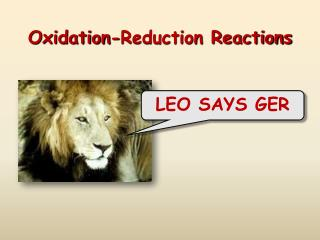Download PresentationOxidation-Reduction Reactions

# Oxidation-Reduction Reactions - PowerPoint PPT PresentationDownload Presentation## Oxidation-Reduction Reactions

- - - - - - - - - - - - - - - - - - - - - - - - - - - E N D - - - - - - - - - - - - - - - - - - - - - - - - - - -
##### Presentation Transcript

1. Oxidation-Reduction Reactions LEO SAYS GER

2. Oxidation and Reduction (Redox) • Electrons are transferred • Spontaneous redoxrxns can transfer energy • Electrons (electricity) • Heat • Non-spontaneous redoxrxns can be made to happen with electricity

3. Oxidation Reduction Reactions(Redox) Each sodium atom loses one electron: Each chlorine atom gains one electron:

4. LEO says GER : Lose Electrons = Oxidation Sodium is oxidized Gain Electrons = Reduction Chlorine is reduced

5. Not All Reactions are Redox Reactions Reactions in which there has been no change in oxidation number are not redoxrxns. Examples:

6. Rules for Assigning Oxidation NumbersRules 1 & 2 • The oxidation number of any uncombined element is zero 2. The oxidation number of a monatomic ion equals its charge

7. Rules for Assigning Oxidation NumbersRules 3 & 4 3.The oxidation number of oxygen in compounds is -2 4. The oxidation number of hydrogen in compounds is +1

8. Rules for Assigning Oxidation Number Rule 5 5. The sum of the oxidation numbers in the formula of a compound is 0 2(+1) + (-2) = 0 H O (+2) + 2(-2) + 2(+1) = 0 Ca O H

9. Rules for Assigning Oxidation NumbersRule 6 6. The sum of the oxidation numbers in the formula of a polyatomic ion is equal to its charge X + 4(-2) = -2 S O X + 3(-2) = -1 N O  X = +5  X = +6

10. Reducing Agents and Oxidizing Agents • The substance reduced is the oxidizingagent • The substance oxidized is the reducingagent Sodium is oxidized – it is the reducing agent Chlorine is reduced – it is the oxidizing agent

11. Trends in Oxidation and Reduction • Active metals: • Lose electrons easily • Are easily oxidized • Are strong reducing agents • Active nonmetals: • Gain electrons easily • Are easily reduced • Are strong oxidizing agents

12. Electrochemical Terminology Electrode: A conductor used to establish contact with a nonmetallic part of a circuit, such as an electrolyte Half-cell: a metal electrode in contact with a solution of its own ions Anode: The electrode where oxidation takes place Cathode: The electrode where reduction takes place

13. Voltaic Cells Anode: negative Cathode: positive Voltaic cells: Electrochemical cells in which a spontaneous redox reaction can be harnessed to produce an electric current.

14. Zinc – Copper Battery

15. Zinc-Carbon Dry Cell Battery

16. Mercury Dry Cell Battery